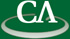## On probabilistic analysis of randomization in hybrid symbolic-numeric algorithms.

### Zhengfeng Yang, Department of Mathematics and Computing Science, NCSU

Wednesday December 5th, 2007, 2:30pm, K9509.

```Abstract:

Algebraic randomization techniques can be applied to hybrid symbolic-numeric
algorithms.  Here we consider the problem of interpolating a sparse rational
function from noisy values.  We develop a new hybrid algorithm based on
Zippel's original sparse polynomial interpolation technique.
We show experimentally that our algorithm can handle sparse polynomials with
large degrees.  We also give a (partial) mathematical justification for
why Zippel's algebraic randomization technique can be used with our
approximate data: the randomly generated non-zero values are expected to be
bounded away from zero.  We show that the random Fourier-like matrices arising
in our algorithm, have the desired rank property in the exact case, and
appear usable numerically.
```